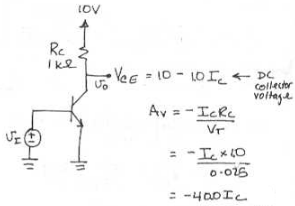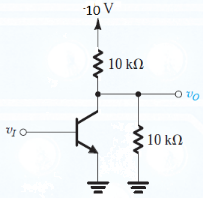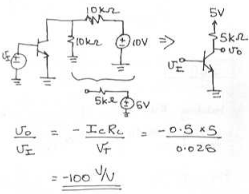Courses

# Test: BJT in Amplifier Design

## 10 Questions MCQ Test Analog Circuits | Test: BJT in Amplifier Design

Description
This mock test of Test: BJT in Amplifier Design for Electronics and Communication Engineering (ECE) helps you for every Electronics and Communication Engineering (ECE) entrance exam. This contains 10 Multiple Choice Questions for Electronics and Communication Engineering (ECE) Test: BJT in Amplifier Design (mcq) to study with solutions a complete question bank. The solved questions answers in this Test: BJT in Amplifier Design quiz give you a good mix of easy questions and tough questions. Electronics and Communication Engineering (ECE) students definitely take this Test: BJT in Amplifier Design exercise for a better result in the exam. You can find other Test: BJT in Amplifier Design extra questions, long questions & short questions for Electronics and Communication Engineering (ECE) on EduRev as well by searching above.
QUESTION: 1

Solution:
QUESTION: 2

### The corresponding maximum input signal permitted is

Solution:

If we assume linear operation right to saturation we can use the gain Av to calculate the maximum input signal. Thus for an output swing ∆ Vo = 0.8 we have
∆ Vi = ∆ Vo / Av = -0.7 / -360 = 1.94 mV.

QUESTION: 3

### (Q.3- Q.5) For the amplifier circuit in Fig. 6.33(a) with Vcc = +10 V, Rc = 1 kΩ and the DC collector bias current equal to Ic Q. Find the voltage gain.

Solution:QUESTION: 4

The maximum possible positive output signal swing as determined by the need to keep the transistor in the active region.

Solution:

Assuming the output voltage Vo = 0.3v is the lowest Vce to stay out of saturation.
Vo = 0.3 = 10 – IcRc
= 10 – IcRc + ∆Vo
∆ Vo = -10 + 0.3 + Ic*1.

QUESTION: 5

The maximum possible negative output signal swing as determined by the need to keep the transistor in the active region.

Solution:

Maximum output voltage before the Transistor is cutoff.
Vce + ∆Vo = Vcc
∆Vo = Vcc – Vce
= 10 – 10 + 10 Ic
= 10 Ic.

QUESTION: 6

The transistor in the circuit below is biased at a dc collector current of 0.5 mA. What is the voltage gain?Solution:QUESTION: 7

For a BJt Vt is 5 V, Rc = 1000 ohm and bias current Ic is 12 mA. The value of the voltage gain is __________

Solution:

Voltage gain is (Ic X Rc ) / Vt.

QUESTION: 8

(Q.8–Q.10) (Q.3- Q.5) For the BJT amplifier circuit with Vcc = +10 V, Rc = 1 kΩ and the DC collector bias current equal to 5 mA,

Q. The value of the voltage gain is _______________

Solution:

The voltage is 400 X Ic where Ic is 5 mA.

QUESTION: 9

The maximum possible positive output signal swing as determined by the need to keep the transistor in the active region.

Solution:

The maximum voltage swing is given by -10 + 0.3 + (Ic X Rc). Putting Ic as 5 mA, we get -4.7 mV.

QUESTION: 10

The maximum possible negative output signal swing as determined by the need to keep the transistor in the active region.

Solution:

It is given by -10 + 10 + (Ic X Rc). Putting Ic as 5 mA we get 5V.